1593542940

# Heap Sort Explained

As you may know, heapsort is also one of the most asked sorting algorithms besides mergesort and quicksort and today we’re going to talk about it and I’ll try my best to explain it in much simpler terms as for a beginner it can be quite complicated more so if one has less knowledge about heaps. But fear not I’ve got your back. So now let’s get into it.

Before moving on to heap sort you need to know about the following terminologies to properly understand the working of heapsort.

1. Heaps
2. Heap Property
3. Heapify

So let’s begin by explaining the terms stated above

# 1. Heaps

Heap data structure is a**_ complete binary tree that follows the heapify property_**. So what’s a complete binary tee you might ask?. A complete binary tree follows these conditions.

• All nodes in every level of the tree are filled except the leaf nodes.
• The nodes are as far left as possible.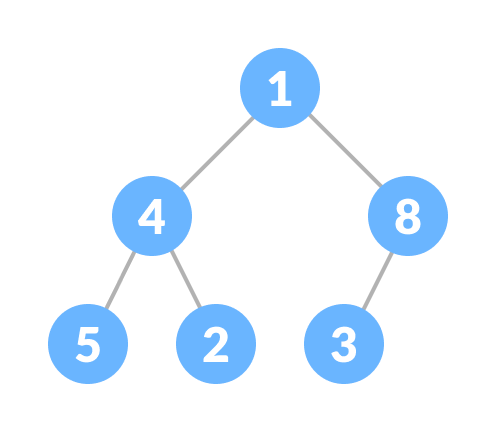Complete Binary Tree

The heap is shown below following the heap property and this is called Max heap. It has the same root and the child nodes but there is a slight difference. The Complete Binay Tree doesn’t care whether it’s root node is smaller or larger but it is of grave importance in case of the heap data structure.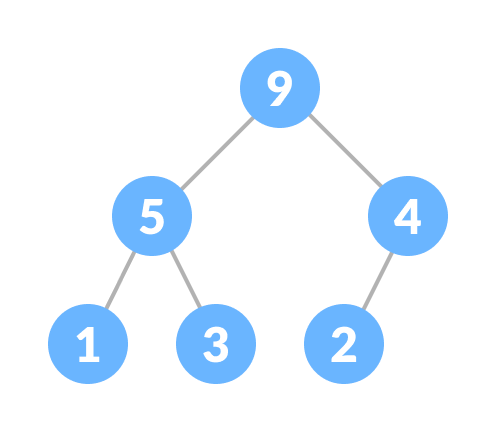Heap

# 2. Heap Property

While creating a heap it is essential to follow the heap property which states as follows:

• The heap where the parent node is always greater than the child nodes and provided that the root node is largest is called a Max-heap.Max Heap

• The heap in which the child node is always greater than it’s child nodes and the root node is the smallest node is called a Min-heap.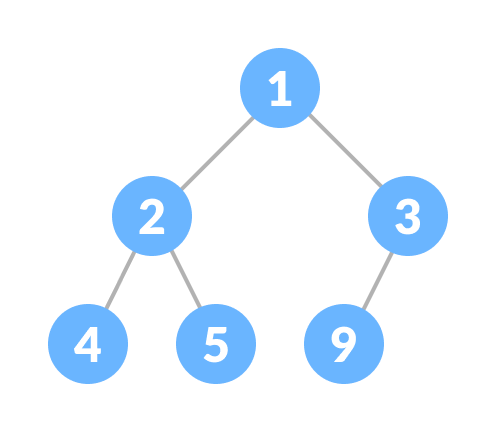Min heap

# 3. Heapify

Heapify is the** process of creating a heap data structure from a binary tree. **Now Heapify is the most important operation on a heap data structure as it helps to maintain the structure of the heap after several operations have been performed on the heap like(insertion, deletion). We will be using this operation a lot and it will come in handy while designing heap sort.

working of heapify is shown below

1. Select an input array.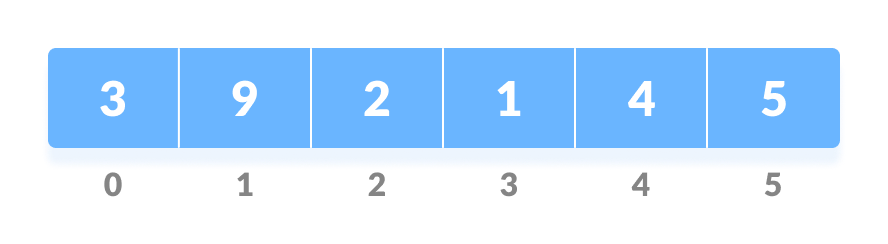Input array

2. Convert this array into a complete binary tree.

Complete binary tree created from the array

3. Create a heap from the binary tree.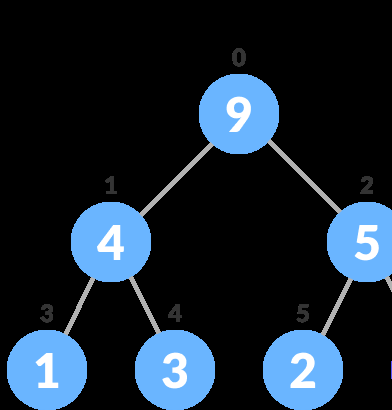After Heapify a max heap is created

Now that we’ve learned about all the important terms let’s start to create heap sort algorithm.

In a heap sort program, we’ll be given an unsorted array and we have to sort it. But heapsort works somewhat different than other sorting algorithms. By different, means the arrangement of each element after operations are performed. In this, the larger elements are sorted first in the last place then followed by the smaller elements, and at last, when there’s one element left that element would be the smallest and the last to go in it’s sorted place.

#sorting #sorting-algorithms #heap #heapsort #sort

## Buddha Community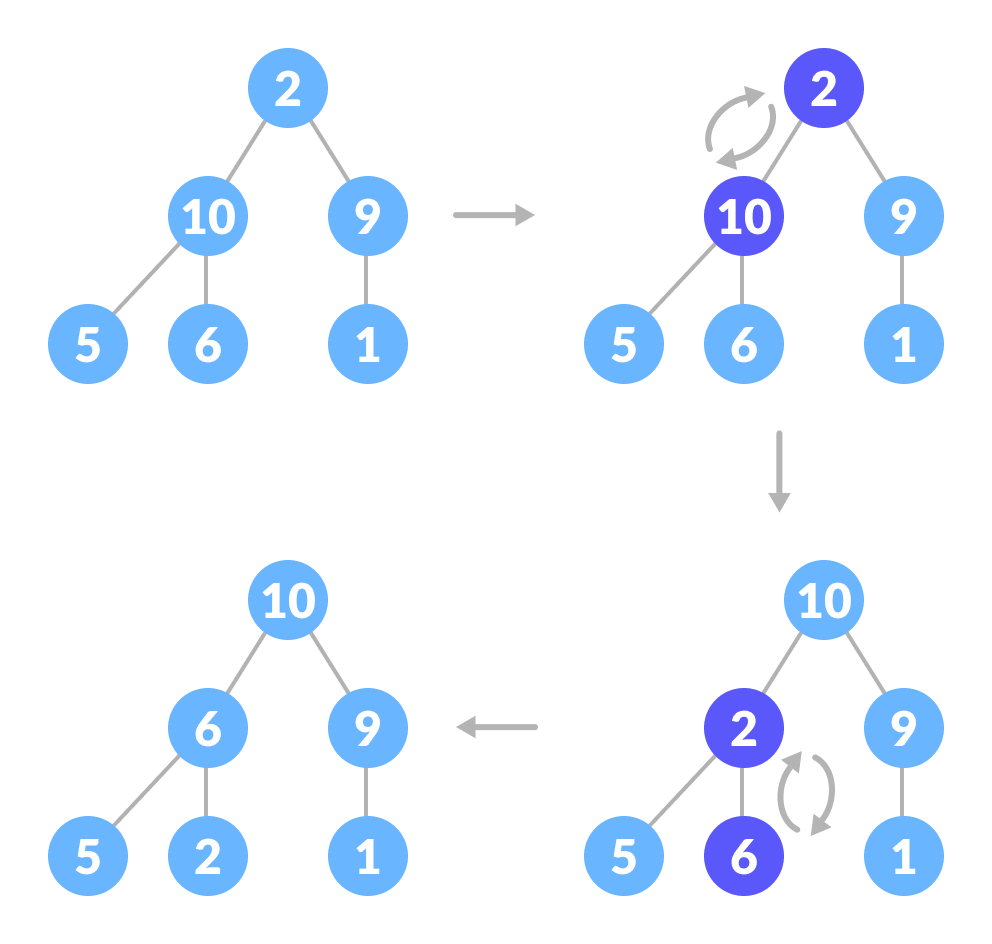1593542940

## Heap Sort Explained

As you may know, heapsort is also one of the most asked sorting algorithms besides mergesort and quicksort and today we’re going to talk about it and I’ll try my best to explain it in much simpler terms as for a beginner it can be quite complicated more so if one has less knowledge about heaps. But fear not I’ve got your back. So now let’s get into it.

Before moving on to heap sort you need to know about the following terminologies to properly understand the working of heapsort.

1. Heaps
2. Heap Property
3. Heapify

So let’s begin by explaining the terms stated above

# 1. Heaps

Heap data structure is a**_ complete binary tree that follows the heapify property_**. So what’s a complete binary tee you might ask?. A complete binary tree follows these conditions.

• All nodes in every level of the tree are filled except the leaf nodes.
• The nodes are as far left as possible.Complete Binary Tree

The heap is shown below following the heap property and this is called Max heap. It has the same root and the child nodes but there is a slight difference. The Complete Binay Tree doesn’t care whether it’s root node is smaller or larger but it is of grave importance in case of the heap data structure.Heap

# 2. Heap Property

While creating a heap it is essential to follow the heap property which states as follows:

• The heap where the parent node is always greater than the child nodes and provided that the root node is largest is called a Max-heap.Max Heap

• The heap in which the child node is always greater than it’s child nodes and the root node is the smallest node is called a Min-heap.Min heap

# 3. Heapify

Heapify is the** process of creating a heap data structure from a binary tree. **Now Heapify is the most important operation on a heap data structure as it helps to maintain the structure of the heap after several operations have been performed on the heap like(insertion, deletion). We will be using this operation a lot and it will come in handy while designing heap sort.

working of heapify is shown below

1. Select an input array.Input array

2. Convert this array into a complete binary tree.

Complete binary tree created from the array

3. Create a heap from the binary tree.After Heapify a max heap is created

Now that we’ve learned about all the important terms let’s start to create heap sort algorithm.

In a heap sort program, we’ll be given an unsorted array and we have to sort it. But heapsort works somewhat different than other sorting algorithms. By different, means the arrangement of each element after operations are performed. In this, the larger elements are sorted first in the last place then followed by the smaller elements, and at last, when there’s one element left that element would be the smallest and the last to go in it’s sorted place.

#sorting #sorting-algorithms #heap #heapsort #sort1603846800

## Merge two sorted arrays in O(1) extra space using Heap

Given two sorted arraysarr[]brr[] of size N, and M, the task is to merge the two given arrays such that they form a sorted sequence of integers combining elements of both the arrays.

Examples:

Input:_ arr[] = {10}, brr[] = {2, 3}_

Output: 2 3 10

Explanation:_ The merged sorted array obtained by taking all the elements from the both the arrays is {2, 3, 10}._

Therefore, the required output is 2 3 10.

Input:_ arr[]__= {1, 5, 9, 10, 15, 20}, brr[] = {2, 3, 8, 13}_

Output:_ 1 2 3 5 8 9 10 13 15 20_

Naive Approach: Refer to Merge two sorted arrays for the simplest approach to merge the two given arrays.

_Time Complexity: _O(N * M)

Auxiliary Space:_ O(1)_

Space Optimized Approach: Refer to Merge two sorted arrays with O(1) extra space to merge the two given arrays without using any extra memory.

Time Complexity:_ O(N * M)_

Auxiliary Space:_ O(1)_

Efficient Space Optimized Approach: Refer to Efficiently merging two sorted arrays with O(1) extra space to merge the two given array without using any extra memory.

Time Complexity:_ O((N + M) * log(N + M))_

Auxiliary Space:_ O(1)_

Heap** – based Approach:** The problem can be solved using Heap. The idea is to traverse arr[] array and compare the value of arr[i] with brr and check if arr[i] is greater than **brr **or not. If found to be true then swap(arr[i], brr[0) and perform the heapify operation on brr[]. Follow the steps below to solve the problem:

• Traverse the array arr[] and compare the value of arr[i] with brr and check if arr[i] is greater than brr or not. If found to be true then swap(arr[i], brr[0) and perform the heapify operation on brr[].
• Finally, sort the array brr[] and print both array.

Below is the implementation of the above approach:

• C++

`// C++ program to implement`

`// the above approach`

`#include <bits/stdc++.h>`

`**using**` `**namespace**` `std;`

`// Function to perform min heapify`

`// on array brr[]`

`**void**` `minHeapify(``**int**` `brr[],` `**int**` `i,` `**int**` `M)`

`{`

`// Stores index of left child`

`// of i.`

`**int**` `left = 2 * i + 1;`

`// Stores index of right child`

`// of i.`

`**int**` `right = 2 * i + 2;`

`// Stores index of the smallest element`

`// in (arr[i], arr[left], arr[right])`

`**int**` `smallest = i;`

`// Check if arr[left] less than`

`// arr[smallest]`

`**if**` `(left < M && brr[left] < brr[smallest]) {`

`// Update smallest`

`smallest = left;`

`}`

`// Check if arr[right] less than`

`// arr[smallest]`

`**if**` `(right < M && brr[right] < brr[smallest]) {`

`// Update smallest`

`smallest = right;`

`}`

`// if i is not the index`

`// of smallest element`

`**if**` `(smallest != i) {`

`// Swap arr[i] and arr[smallest]`

`swap(brr[i], brr[smallest]);`

`// Perform heapify on smallest`

`minHeapify(brr, smallest, M);`

`}`

`}`

`// Function to merge two sorted arrays`

`**void**` `merge(``**int**` `arr[],` `**int**` `brr[],`

`**int**` `N,` `**int**` `M)`

`{`

`// Traverse the array arr[]`

`**for**` `(``**int**` `i = 0; i < N; ++i) {`

`// Check if current element`

`// is less than brr`

`**if**` `(arr[i] > brr) {`

`// Swap arr[i] and brr`

`swap(arr[i], brr);`

`// Perform heapify on brr[]`

`minHeapify(brr, 0, M);`

`}`

`}`

`// Sort array brr[]`

`sort(brr, brr + M);`

`}`

`// Function to print array elements`

`**void**` `printArray(``**int**` `arr[],` `**int**` `N)`

`{`

`// Traverse array arr[]`

`**for**` `(``**int**` `i = 0; i < N; i++)`

`cout << arr[i] <<` `" "``;`

`}`

`// Driver Code`

`**int**` `main()`

`{`

`**int**` `arr[] = { 2, 23, 35, 235, 2335 };`

`**int**` `brr[] = { 3, 5 };`

`**int**` `N =` `**sizeof**``(arr) /` `**sizeof**``(arr);`

`**int**` `M =` `**sizeof**``(brr) /` `**sizeof**``(brr);`

`// Function call to merge`

`// two array`

`merge(arr, brr, N, M);`

`// Print first array`

`printArray(arr, N);`

`// Print Second array.`

`printArray(brr, M);`

`**return**` `0;`

`}`

Output:

``````2 3 5 23 35 235 2335
``````

Time Complexity:_ O((N + M) * log (M))_

Auxiliary Space:_ O(1)_

Efficient Approach: Refer to merge two sorted arrays to efficiently merge the two given arrays.

_Time Complexity: _O(N + M)

Auxiliary Space:_ O(N + M)_

Attention reader! Don’t stop learning now. Get hold of all the important DSA concepts with the DSA Self Paced Course at a student-friendly price and become industry ready.

#arrays #divide and conquer #heap #mathematical #sorting #amazon #amdocs #array-merge #brocade #goldman sachs #heap sort #juniper networks #priority-queue #snapdeal1599235200

## C++ Heap Sort Example | Heap Sort Program In C++

C++ Heap Sort is a sorting method based on comparisons between the elements. The heapsort is a comparison based sorting technique based on a Binary Heap data structure. The sorting method works on the principle of binary heap data structure.

The binary heap data structure is much similar to the binary tree. A binary heap is a binary tree in which the elements are stored in a particular tree-like structure. In this, the parent node is either greater (or smaller) than the values stored in the child nodes. According to this, the heaps are either called max heap or min-heap, respectively.

We have already covered  QuickSort,  MergeSort, and  BubbleSort in C++ in this blog.

#c++ #c++ heap sort

1599645721

## Bubble Sort Algorithm Demystified

As Bubble Sort represents the basic foundations of sorting, we’ve tried to demystify this sorting algorithm.

#bubble-sort #sorting #sorting-algorithm #array #practice1624456980

## How to Sort a HashMap by Key in Java

In this tutorial, we’ll take a look at how to sort a HashMap by key in Java.

Let’s go ahead and create a simple `HashMap`:

``````Map<String, Integer> unsortedMap = new HashMap();

unsortedMap.put("John", 21);
unsortedMap.put("Maria", 34);
unsortedMap.put("Mark", 31);
unsortedMap.put("Sydney", 24);

unsortedMap.entrySet().forEach(System.out::println);
``````

We’ve got `String`s as keys, and `Integer`s as values. Most of the time, you’ll encounter `Integer`s or `String`s as keys, and custom objects, `String`s or `Integer`s as values. We’ll want to sort this `HashMap`, based on the `String` keys.

`HashMap`don’t guarantee to maintain the order of its elements in any case. The order can change through time, and they most definitely won’t be printed back in the order of insertion:

``````John=21
Mark=31
Maria=34
Sydney=24
``````

If you re-run this program, it’ll keep this order, since `HashMap`s order their elements into bins, based on the hash value of the keys. When printing values from a `HashMap`, its contents are printed sequentially, so the results will stay the same if we re-run the program multiple times.

#java #how to sort a hashmap by key in java #hashmap #key in java #sort #sort a hashmap by key in java